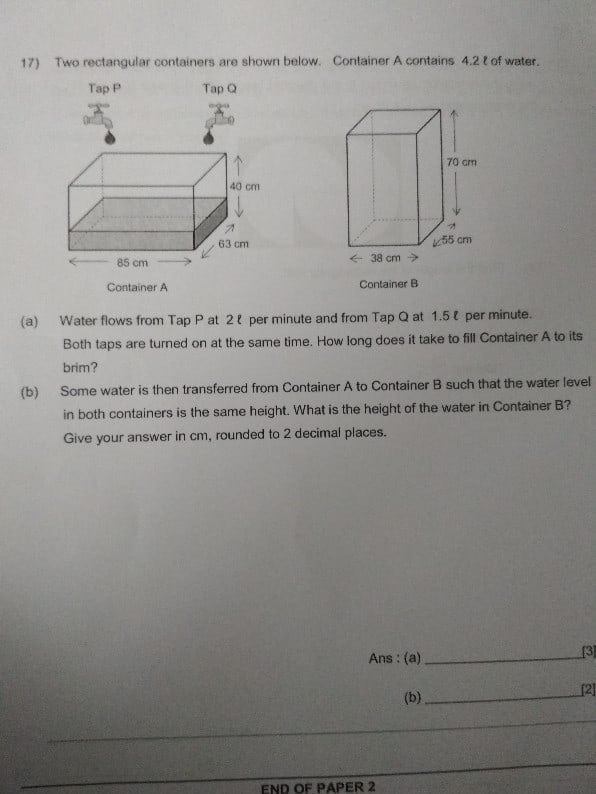# QuestionI don’t know how to do the whole question

Important reminder:

• Volume = Height x Width x Depth
• 1 L = 1000 cm3

The container A holds water up to 85 x 63 x 40 = 214 200 cm3

214 200 cm3 = 214.2 l

Therefore, the additional water container A can hold is 214.2 – 4.2 = 210 l

If tap P and Q are running at the same time, there will be (2 l + 1.5 l) per minute

a> 210 ÷ 3.5 = 60 min

The total volume of the water is 214 200 cm3

The sum of the bottom area of the two containers is 85 x 63 + 38 x 55 = 5355 + 2099 = 7445 cm2

b> 214 200 ÷ 7445 = 28.77 cm

0 Replies 0 Likes ✔Accepted Answer